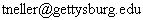CS 371 Introduction to Artificial Intelligence Homework #9

Due: Thursday, 11/9
1. Learning Boolean Functions with Back-Propagation:  Implement back-propagation as described in Section 19.4 of our text.  Also implement the momentum variant described in class.  Use the sigmoid function as your activation function.  No extra thresholding units will be necessary.  An implementation of a BooleanFunction class and an interface for your neural network implementation is provided here.  Javadoc documentation can be found here.  Details of what you should implement are given in the comments of interface MultilayerFeedForwardNeuralNetwork.  Training of the network can, of course, terminate early if the network has converged to correct weights.*
2. Varying arity: Create simple neural networks with n inputs, 2^n hidden units, and 1 output unit.  Such networks are capable of learning any boolean function.  Your first experiments will seek to gain understanding of how many training epochs are necessary to learn random boolean functions.  Do not use momentum for this experimentation, and use a  learning rate of 0.1.  Do simple analysis of the epoch data (mean, median) and plot a histogram with 4-10 intervals for three different arity: n=3,4,5.  Be sure to set an epoch limit of at least 1000 in case.  What do you observe?
3. Varying learning rate: Continuing with the previous network configuration, let n=3.  Vary the learning rate and repeat the analysis of the previous question.

4. At least learning rate values (.25, .05, .1, .2, .4).  What do you observe?
5. Varying momentum: Continuing with the previous network, use a fixed learning rate of 0.1.  Vary the momentum constant and repeat the analysis of the previous question.  Test at least four values between 0.0 (no momentum) and 1.0.  What do you observe?
6. Independent experimentation:  Design an experiment of your own to gain further understanding of some aspect of back-propagation.  This experiment can be a refinement of one of the previous three experiments, with a range of values providing a clearer picture.  You might instead seek to vary two parameters to see if there's a dependency.  You might think of something completely different.  This is an important opportunity to think as a researcher: (1) pose an interesting question, (2) design an experiment to gain insight, (3) execute the experiment, (4) analyze the results, and (5) share your findings.

* NOTE: What it means for your neural network to converge to correct weights: If, for all boolean true/false inputs converted to 1.0/0.0, the network's output is >= 0.5 if and only if the correct output is true, then the network has converged.  In other words the boolean expression (output >= 0.5) should be equivalent to the desired boolean output.  However, this does not mean that the network output should be rounded to the nearest integer.  0.0 and 1.0 are still the target values and the non-target values are still needed for error.  A network can converge and still have error.

(c) 2000 Todd Neller